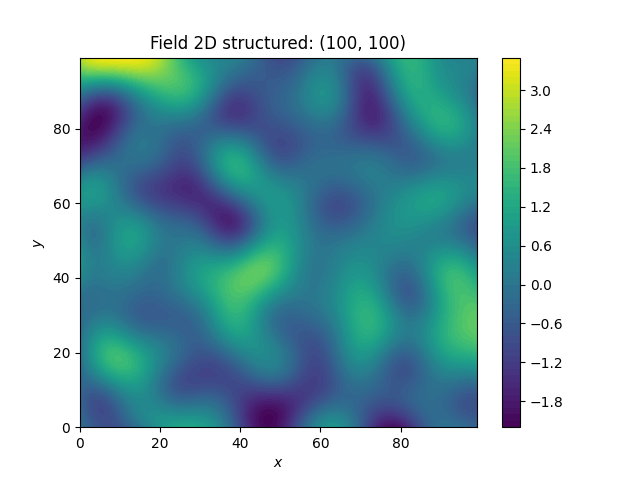# A Very Simple Example¶

We are going to start with a very simple example of a spatial random field with an isotropic Gaussian covariance model and following parameters:

• variance• correlation lengthFirst, we set things up and create the axes for the field. We are going to need the SRF class for the actual generation of the spatial random field. But SRF also needs a covariance model and we will simply take the Gaussian model.

import gstools as gs

x = y = range(100)


Now we create the covariance model with the parametersandand hand it over to SRF. By specifying a seed, we make sure to create reproducible results:

model = gs.Gaussian(dim=2, var=1, len_scale=10)
srf = gs.SRF(model, seed=20170519)


With these simple steps, everything is ready to create our first random field. We will create the field on a structured grid (as you might have guessed from the x and y), which makes it easier to plot.

field = srf.structured([x, y])
srf.plot()Wow, that was pretty easy!

Total running time of the script: ( 0 minutes 1.325 seconds)

Gallery generated by Sphinx-Gallery